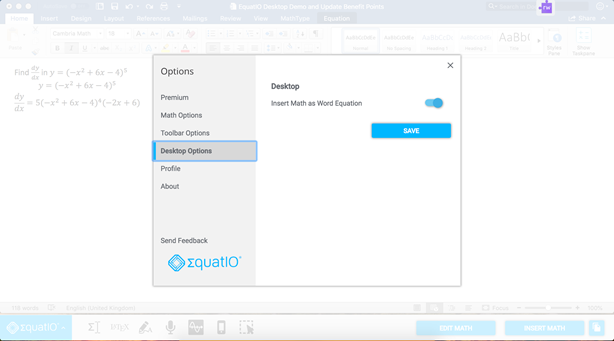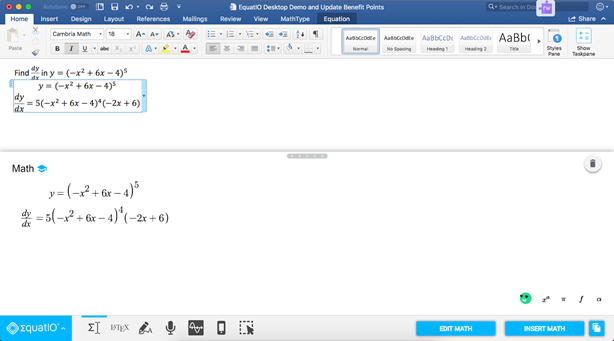# EquatIO is Getting Smarter for Higher Ed

We have released a new update to EquatIO for Windows and EquatIO for Mac, which enables Microsoft Word users to edit equations built with MathType and the Microsoft Equation Editor directly with EquatIO and even reinsert them as Microsoft Word equations.

Math departments in Higher Ed typically use MathType or the Microsoft Equation Editor as their equation editor types when creating course documents in Microsoft Word. This is because:

• The equations look more professional than inserted images
• They can put the math inline with the rest of their text
• It is more apparent that the math is editable due to the box around the math

However, creating and editing math with pallete-based editors can be very cumbersome and time consuming.

That’s where the latest update to EquatIO for Windows and EquatIO for Mac* comes in. Users can now go to the Desktop Options tab within EquatIO and turn on ‘Insert Math as Word Equation’. With this feature turned on, any math that is created with EquatIO will be inserted into the Word Document as a Microsoft Equation.What’s more, if you ever need to edit an equation that was built with MathType or the Microsoft Equation Editor, you can now edit it with EquatIO just like you would any other EquatIO item. Simply select the equation in the Word Doc and hit ‘Edit Math’ in the EquatIO toolbar. This will pull the equation into EquatIO for quick and easy updates.This is also a great feature for Disability Services departments who often have to come up with complicated workflows to convert math equations built with these equation editor types into MathML, a computer language that screenreaders can read aloud. Now, thanks to EquatIO’s support for MathType and Microsoft Equation Editor, they can convert math more quickly to make it accessible for their students.

* EquatIO for Mac users will automatically be prompted to install the new version of EquatIO the next time they open the application and will need to be on the latest version of Microsoft Word. EquatIO for Windows users will need to visit this link to install the new version.

Let us know what you think about the new updates to EquatIO for Windows and EquatIO for Mac in the comments section below.